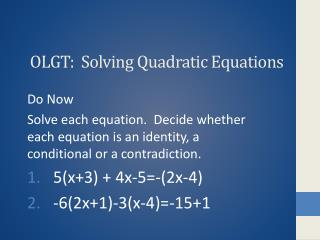DownloadDownload PresentationDownload Presentation- - - - - - - - - - - - - - - - - - - - - - - - - - - E N D - - - - - - - - - - - - - - - - - - - - - - - - - - -
##### Presentation Transcript

1. OLGT: Solving Quadratic Equations Do Now Solve each equation. Decide whether each equation is an identity, a conditional or a contradiction. 5(x+3) + 4x-5=-(2x-4) -6(2x+1)-3(x-4)=-15+1

2. Using the Zero-Factor Property • A quadratic equation written in standard form is • Ax2+bx+c = 0, where a, b, c are real numbers and a can not equal zero. • You can solve them by using one of the three methods • Zero-factor Property • Square Root Property • Quadratic Formula

3. Zero-Factor Property • Solve 6x2+7x=3 • First put in standard form • 6x2+7x-3=0 • Then factor • (3x-1)(2x-3)=0

4. Zero-Factor Property • Apply the zero-factor property • 3x-1=0 or 2x+3=0 • 3x=1 2x=-3 • X=1/3 x=-3/2 • Check • 6(1/3)2+7(1/3)=3 and • 6(-3/2)2+7(-3/2)=3

5. Using the Square Root Property • Solve the quadratic equations x2=17 2. (x-4)2=12 X= x-4 = x=4 x= x=4

6. Solve by the zero-property • -6x2+7x =10 • -6x2+7x -10 = 0 • -1(6x2-7x +10)=0 • -1(6x+5)(x-2) =0 • 6x-5=0 or x-2 =0 • 6x=5 • X=5/6 or x=2

7. Square Root Property • (x-7)2=24 • X-7 = • X =7 • X=7 • 7

8. Homework • Page 441 # 33-44